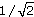Science, Maths & Technology

### OU short coursesSound for music technology: An introduction

Start this free course now. Just create an account and sign in. Enrol and complete the course for a free statement of participation or digital badge if available.

# 6.3 Root-mean-square amplitude

One drawback of the amplitude as I have defined it is that although it allows the relative sizes of sine waves to be compared, it does not give a good idea of what a sine wave can deliver in absolute terms. For instance, a sine wave with an amplitude of 10 volts has twice the amplitude of one with an amplitude of 5 volts. But is a power source that delivers a sine wave with an amplitude of 10 volts as powerful as, say, a 10 volt battery? Could you use it to drive a bulb and get the same illumination? The answer is ‘no’, and the discrepancy is due to the fluctuating nature of the sine wave, which for most of each cycle is well below its maximum value. It is thus often useful to specify the magnitude of a sine wave in a way that facilitates direct comparison with a non-oscillatory source of energy. One benefit of this is that it enables us to say how big a non-oscillatory source would be needed to deliver the same energy as the sine wave delivers in a particular length of time.

A tempting solution might simply be to use the average value of the sine wave over several cycles, but this turns out not to be useful. For instance, the average value of the sine wave in Figure 16 is zero, but this sine wave would certainly be capable of transferring energy.

The solution to the problem is found in the concept of the root-mean-square amplitude (r.m.s. amplitude) of a sine wave, which is mainly used in the context of electrical sine waves. You can think of this as an alternative way of specifying how big a sine wave is, but with the advantage of allowing direct comparison with a non-oscillating source of energy. The root mean square is a kind of average, but it is derived by calculating the average power of a sine wave.

The root-mean-square amplitude of a sine wave is its amplitude multiplied by a factor of approximately 0.71. (The actual value is, which to five decimal places is 0.70711.) Thus, a sine wave with an amplitude of 10 volts has an r.m.s. amplitude of (approximately) 0.71 ×10 volts, which is 7.1 volts. It therefore conveys energy at the same rate as a steady 7.1 volt source, other things being equal. The r.m.s. amplitude of a sine wave is proportional to the amplitude as I defined it earlier. Doubling one doubles the other; tripling one triples the other; and so on.

## Activity 22 (Self-Assessment)

A sine wave has a peak-to-peak amplitude of 2 volts.

• (a) What is its amplitude?

• (b) What is its r.m.s. amplitude?

• (c) What steady source of voltage (e.g. a battery) would deliver energy at the same rate, other things being equal?